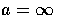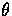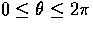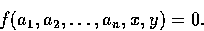Next: References Up: Computer Vision IT412 Previous: Classical Feature Detection

# The Hough Transform

The Hough transform is a method that, in theory, can be used to find features of any shape in an image. In practice it is only generally used for finding straight lines or circles. The computational complexity of the method grows rapidly with more complex shapes.

Assume we have some data points in an image which are perhaps the result of an edge detection process, or boundary points of a binary blob. We wish to recognise the points that form a straight line.

Consider a point (xi, yi) in the image. The general equation of a line is

y = ax + b.

There are infinitely many lines that pass through this point, but they all satisfy the condition

yi = axi + b

for varying a and b.

We can rewrite this equation as

b = -xia + yi,

and plot the variation of a and b.

If we divide parameter space into a number of discrete accumulator cells we can collect `votes' in a b space from each data point in x y space. Peaks in a b space will mark the equations of lines of co-linear points in x y space.

However, we have a problem with using y = ax + b to represent lines when the line is vertical. In that caseand our parameter space is unbounded (we would need a very large computer to store our parameter accumulator array!)

An alternative representation of a line is given bywhere r is the distance of the line from the origin and theta is the angle between this perpendicular and the x-axis. Our parameter space is now inand r, whereand r is limited by the size of the image.

As before, peaks in the accumulator array mark the equations of significant lines.

In theory any kind of curve can be detected if you can express it as a function of the formFor example a circle can be represented as

(x - a)2 + (y - b)2 - r2 = 0.

One then has to work in n dimensional parameter space (three dimensional space for a circle).

This model has three parameters: two parameters for the centre of the circle and one parameter for the radius of the circle. If the gradient angle for the edges is available, then this provides a constraint that reduces the number of degrees of freedom and hence the required size of the parameter space. The direction of the vector from the centre of the circle to each edge point is determined by the gradient angle, leaving the value of the radius as the only unknown parameter.

Thus, the parametric equations for a circle in polar coordinates are

 x = a + r cosq
and
 y = b + r sinq.
Solving for the parameters of the circle we obtain the equations
 a = x - r cosq
and
 b = y - r sinq.
Now given the gradient angle q at an edge point (x,y), we can compute cosq and sinq. Note that these quantities may already be available as a by-product of edge detection. We can eliminate the radius from the pair of equations above to yield
 b = a tanq- x tanq+ y.
Then the Hough Transform algorithm for circle fitting can be described as follows.

### Circle Fitting Algorithm

1. Quantise the parameter space for the parameters a and b.
2. Zero the accumulator array M(a,b).
3. Compute the gradient magnitude G(x,y) and angle q(x,y).
4. For each edge point in G(x,y), increment all points in the accumulator array M(a,b) along the line
 b = a tanq- x tanq+ y.
5. Local maxima in the accumulator array correspond to centres of circles in the image.Next: References Up: Computer Vision IT412 Previous: Classical Feature Detection
Robyn Owens
10/29/1997